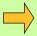Shakuntala Devi was asked to find the 23rd root of a 201 digit number. She found the answer in 50 seconds. After a lengthy program was written and 13,000 instructions were fed into a computer the machine gave the same answer, but it took a full minute.

Shakuntala Devi refers to her calculating powers as her "God-given gift". She lives in Calcutta and often travels, giving demonstrations of the talents.

Chapter 4

The First by the First and the Last by the Last

This formula provides us with two very simple and very quick checks on our calculations and another special type of multiplication. A third checking device is also shown in this chapter.

Check 1: The First by the FirstExample 1

32 × 41 is approximately 1200

by multiplying the first figure of each number together we find that 32 × 41 is approximately 30 × 40, which is 1200.
We also know that it will be a little over 1200 because 32 and 41 are both over 30 and 40.Example 2

641 × 82 is approximately 50,000

since 600 × 80 = 48,000 and we know the answer will be more than this we can say the answer is about 50,000.Example 3

39 × 61 is approximately 2400

here we can think of 39 as 41 (that is 40 - 1) so that the first by the first gives 40 × 60 = 2400.Example 4

383 × 887 is approximately 300,000

400 × 900 = 360,000 and the answer must be below this because both 400 and 900 are above the original numbers, so we can say 300,000.

Thus we see that The First by the First gives us the first figure of the answer; and the number of figures in the answer is also evident.

Check 2: The Last by the LastExample 5

72 × 83 ends in 6

by multiplying the last figure of each number together we get the last figure of the answer: 2 × 3 = 6.Example 6

383 × 887 ends in 1

since 3 × 7 = 21, which ends with a 1.Example 7

So if we are finding 33 × 8 by doubling 33 three times, and we arrive at 132 but we lose track of how many times we have doubled, we note that the last figure of 33 × 8 must be 4 and that 132 cannot therefore be the answer. But doubling once more to 264 we are now confident that we have the correct answer.

Question Comment !
 Tutorial Title Question Title No Questions 0 Questions Asked 0 Skipped Questions 0 Correctly Answered 0 Wrongly Answered 0 Total Question Attempts 0 Current Question Attempts 0

Skip Question

Reset Test

Halt Test

 No Questions 0 Questions Asked 0 Skipped Questions 0 Correctly Answered 0 Wrongly Answered 0 Total Question Attempts 0

Time Taken

CloseExercise A

Check 3: The Digit Sum Check

This is another checking device which can be very useful and which comes under the Vedic formula The Product of the Sum is the Sum of the Products.

Every number, no matter how long, can be reduced to a single figure, called its digit sum, by adding the digits in the number and then adding again if necessary.

So for 43 the digit sum is 7 since 4+3 = 7.
Also for 47: 47 = 11 = 2.
And 876 = 21 = 3.

To check a multiplication sum the above Vedic formula reads: The Product of the Digit Sums is the Digit Sum of the Products. So if we have the sum:Example 8

74 × 76 = 5624

we reduce 74, 76 and 5624 to their digit sums: 74 = 11 = 2,
76 = 13 = 4,
5624 = 17 = 8.

Then replacing the original sum with these digit sums we get 2×4 = 8, which is true, and therefore supports our answer, 5624.Example 9

Similarly 24 × 26 = 624 becomes 6 × 8 = 3.
This is also true in digit sums because 6 × 8 = 48 = 12 = 3.Example 10

This device can also be used for other types of sum.

To check 88 + 77 = 165 for example, the sum becomes 7+5 = 3 in digit sums, which is correct.

This digit sum check does not detect certain errors however: if we wrote
88+77 = 156 the digit sum check would be the same as above even though the answer is wrong. However in this case The Last by the Last tells us that the answer certainly is wrong since it must end with 5.

Multiplying Numbers Whose Last Figures are the Same and Whose First Figures Add up to 10

This complements the last multiplication type in the previous chapter in which the first figures are the same and the last add up to 10.Example 11

27 × 87 = 23/49

The conditions are satisfied here as 2 + 8 = 10 and both numbers end in 7.

So we multiply the first figure of each number together and
add the last figure: 2 × 8 = 16, 16 + 7 = 23 which is the first part of the answer.
Multiplying the last figures together: 7×7 = 49: which is the last part of the answer.Example 12

Similarly 69 × 49 = 3381

in which 33 = 6×4 + 9, and 81 = 9×9.Exercise B

Optional extra question to challenge the advanced student

1. Can you find out how to extend the method to the following two cases?
1. multiplying numbers in which the first figures add up to 10 and the last two figures are the same: e.g. 212 × 812,

2. multiplying numbers in which the the first two figures add up to 100 and the last two figures are the same e.g. 987 × 027.